### Home > MC2 > Chapter 5 > Lesson 5.1.3 > Problem5-38

5-38.
1. Simplify each expression. Homework Help ✎

1. 1.2 – 0.8

2. –4 – (–2)

3.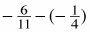4.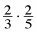5. 0.6 · 8

6.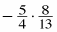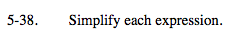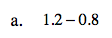Remember to line up the decimal points when adding or subtracting numbers with decimals.

0.4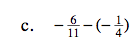Find a common denominator.

See part (b).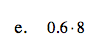When you multiply numbers with decimals, you do not necessarily have to line up the decimal points.

4.8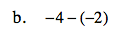When you subtract a negative, you add its opposite.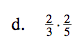Multiply the numerators together and the denominators together.

$\frac{4}{15}$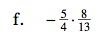Remember, a negative number multiplied by a positive number is always a negative number.